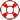Standard:

Description:

Standard:

Math.5.NBT.7 or 5.NBT.B.7

Description:

Add, subtract, multiply, and divide decimals to hundredths, using concrete models or drawings and strategies based on place value, properties of operations, and/or the relationship between addition and subtraction; relate the strategy to a written method and explain the reasoning used.5th Grade Math - Powers of 10 Lesson